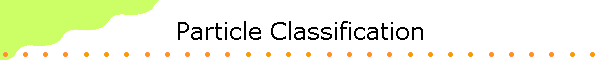The four fundamental interactions or forces that govern the behavior of elementary particles are listed below.

• The strong force  (It holds the nucleus together.)
• The electromagnetic force  (It causes interactions between charges.)
• The weak force  (It causes beta decay.)
• The gravitational force  (It causes interaction between states with energy.)

A given particle may not necessarily be subject to all four interactions.  Neutrinos, for example, experience only the weak and gravitational interaction.

The fundamental particles may be classified into groups in several ways.  First, all particles are classified into fermions, which obey Fermi-Dirac statistics and bosons, which obey Bose-Einstein statistics.  Fermions have half-integer spin, while bosons have integer spin.  All the fundamental fermions have spin 1/2.  Electrons and nucleons are fermions with spin 1/2.  The fundamental bosons have mostly spin 1.  This includes the photon.  The pion has spin 0, while the graviton has spin 2.  There are also three particles, the W+, W and Z0 bosons, which are spin 1.  They are the carriers of the weak interactions.

We can also classify the particles according to their interactions.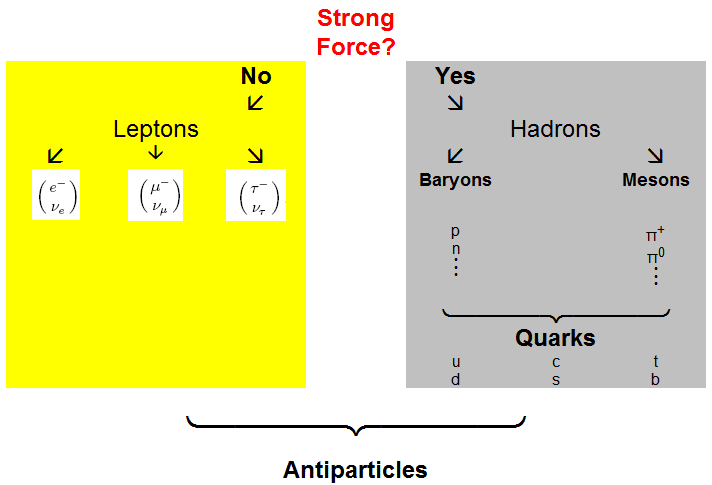The electron and the neutrino are members of a family of leptons.  Originally leptons meant "light particles", as opposed to baryons, or heavy particles, which referred initially to the proton and neutron.  The pion, or pi-meson, and another particle called the muon or mu-meson, were called mesons, or medium-weight particles, because their masses, a few hundred times heavier than the electron but six times lighter than a proton, were in the middle.  But that distinction turned out not to be very useful.  We now recognize the muon to be almost the same as an electron, and the leptons now consist of three "generations" of pairs of particles,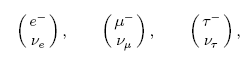with the heaviest of these, the tau lepton τ, being almost twice as massive as the proton

The leptons are distinguished from other particles called hadrons in that leptons do not participate in strong interactions.  The bottom lepton in each of the three "doublets" shown above is not only neutral, but also has a very small mass.  Neutrinos had been considered massless for many years, but more recent experiments have shown their mass to be non-zero.

Table of leptons

particle associated neutrino
Name

Charge
(e)
Mass
(MeV)
Name Charge
(e)
Mass
(MeV)
Electron (e-) -1 0.511 Electron neutrino (νe) 0 < 0.000003
Muon (μ-) -1 105.6 Muon neutrino (νμ) 0 < 0.19
Tau (τ-) -1 1777 Tau neutrino (ντ) 0 < 18.2

Hadrons are strongly interacting particles.  They are divided into baryons and mesons.  The baryons are a class of fermions, including the proton and neutron, and other particles which in a decay always produce another baryon, and ultimately a proton.  The mesons, are bosons.  In addition to the pion, there are other spin 0 particles, four kaons and two eta mesons, and a number of spin one hadrons, including the three rho mesons, which like the pion come in charges 1 and 0.  Mesons can decay without necessarily producing other hadrons.

Table of some baryons

Particle

Symbol

Quark
Content
Mass
MeV/c2
Mean
Decays to

Proton p uud 938.3 Stable Unobserved
Neutron n ddu 939.6 885.7±0.8 p + e- + νe
Delta Δ++ uuu 1232 6×10-24 π+ + p
Delta Δ+ uud 1232 6×10-24 π+ + n or π0 + p
Delta Δ0 udd 1232 6×10-24 π0 + n or π- + p
Delta Δ- ddd 1232 6×10-24 π- + n
Lambda Λ0 uds 1115.7 2.60×10-10 π- + p or πo + n
Sigma Σ+ uus 1189.4    0.8×10-10 π0 + p or π+ + n
Sigma Σ0 uds 1192.5 6×10-20 Λ0 + γ
Sigma Σ- dds 1197.4 1.5×10-10 π- + n
Xi Ξ0 uss 1315 2.9×10-10 Λ0 + π0
Xi Ξ- dss 1321 1.6×10-10 Λ0 + π-
Omega Ω- sss 1672 0.82×10-10     Λ0 + K- or Ξ0 + π-

Table of some mesons

Particle

Symbol

Anti-
particle
Quark
Content
Mass
MeV/c2
Mean
Principal
decays
Charged Pion π+ π ud 139.6 2.60×10-8 μ+ + νμ
Neutral Pion π0 Self uu - dd 135.0 0.84×10-16
Charged Kaon K+ K$\mathrm{u\bar{s}}$ 493.7 1.24×10-8 μ+ + νμ or π+ + π0
Neutral Kaon K0 K0 ds 497.7
Eta η Self uu + dd - 2ss 547.8 5×10-19
Eta Prime η' Self uu + dd + ss 957.6 3×10-21

Each elementary particle is associated with an antiparticle with the same mass and opposite charge.  Some particles, such as the photon, are identical to their antiparticle.  Such particles must be neutral, but not all neutral particles are identical to their antiparticle.  Particle-antiparticle pairs can annihilate each other if they are in appropriate quantum states, releasing an amount of energy equal to twice the rest energy of the particle.  They can also be produced in various processes, if enough energy is available.  The minimum amount of energy needed is twice the rest energy of the particle, if momentum conservation allows the particle-antiparticle pair to be produced at rest.  Most often the antiparticle is denoted by the same symbol as the particle, but with a line over the symbol.   For example, the antiparticle of the proton p, is denoted by p.

Protons and neutrons are made of still smaller particles called quarks.  At this time it appears that the two basic constituents of matter are the leptons and the quarks.  There are believed to be six types of each.  Each quark type is called a flavor, there are six quark flavors.  Each type of lepton and quark also has a corresponding antiparticle, a particle that has the same mass but opposite electrical charge and magnetic moment.  An isolated quark has never been found, quarks appear to almost always be found in pairs or triplets with other quarks and antiquarks.  The resulting particles are the hadrons, more than 200 of which have been identified.  Baryons are made up of 3 quarks, and mesons are made up of a quark and an anti-quark.  Baryons are fermions and mesons are bosons.  Two theoretically predicted five-quark particles, called pentaquarks, have been produced in the laboratory.  Four- and six-quark particles are also predicted but have not been found.

The six quarks have been named up, down, charm, strange, top, and bottom.  The top quark, which has a mass greater than an entire atom of gold, is about 35 times more massive than the next biggest quark and may be the heaviest particle nature has ever created.  The quarks found in ordinary matter are the up and down quarks, from which protons and neutrons are made.  A proton consists of two up quarks and a down quark, and a neutron consists of two down quarks and an up quark.  The pentaquark consists of two up quarks, two down quarks, and the strange antiquark.  Quarks have fractional charges of one third or two thirds of the basic charge of the electron or proton.  Particles made from quarks always have integer charge.

Table of Quarks

Name

Symbol

Charge
(e)
Spin

Mass
MeV/c2
Strangeness

Baryon
number
Lepton
number
up u +2/3 1/2 1.7-3.3 0 1/3 0
down d -1/3 1/2 4.1-5.8 0 1/3 0
strange s -1/3 1/2 101 -1 1/3 0
charm c +2/3 1/2 1270 0 1/3 0
bottom b -1/3 1/2 4190-4670 0 1/3 0
top t +2/3 1/2 172000 0 1/3 0

In the current theory, known as the Standard Model there are 12 fundamental matter particle types and their corresponding antiparticles.  In addition, there are gluons, photons, and W and Z bosons, the force carrier particles that are responsible for strong, electromagnetic, and weak interactions respectively.  These force carriers are also fundamental particles.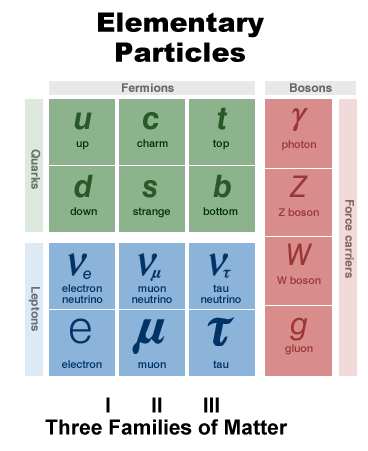All we know is that quarks and leptons are smaller than 10-19 meters in radius.  As far as we can tell, they have no internal structure or even any size.  It is possible that future evidence will, once again, show our understanding to be incomplete and demonstrate that there is substructure within the particles that we now view as fundamental.

The Discovery of Elementary Particles

The first subatomic particle to be discovered was the electron, identified in 1897 by J. J. Thomson.  After the nucleus of the atom was discovered in 1911 by Ernest Rutherford, the nucleus of ordinary hydrogen was recognized to be a single proton.  In 1932 the neutron was discovered.  An atom was seen to consist of a central nucleus containing protons and neutrons, surrounded by orbiting electrons.  However, other elementary particles not found in ordinary atoms immediately began to appear.

In 1928 the relativistic quantum theory of P. A. M. Dirac predicted the existence of a positively charged electron, or positron, which is the antiparticle of the electron.  It was first detected in 1932.  Difficulties in explaining beta decay led to the prediction of the neutrino in 1930, and by 1934 the existence of the neutrino was firmly established in theory, although it was not actually detected until 1956.  Another particle was also added to the list, the photon, which had been first suggested by Einstein in 1905 as part of his quantum theory of the photoelectric effect.

The next particles discovered were related to attempts to explain the strong interactions, or strong nuclear force, binding nucleons together in an atomic nucleus.  In 1935 Hideki Yukawa suggested that a meson, a charged particle with a mass intermediate between those of the electron and the proton, might be exchanged between nucleons.  The meson emitted by one nucleon would be absorbed by another nucleon.  This would produce a strong force between the nucleons, analogous to the force produced by the exchange of photons between charged particles interacting through the electromagnetic force.  It is now known that the strong force is mediated by the gluon.  The following year a particle of approximately the required mass, about 200 times that of the electron, was discovered and named the mu-meson, or muon.  However, its behavior did not conform to that of the theoretical particle.  In 1947 the particle predicted by Yukawa was finally discovered and named the pi-meson, or pion.  Both the muon and the pion were first observed in cosmic rays.  Further studies of cosmic rays turned up more particles.  By the 1950s these elementary particles were also being observed in the laboratory as a result of particle collisions in particle accelerators.

By the early 1960s over 30 "fundamental particles" had been found.  A rigorous way of classifying them was needed.  Were there any symmetries or patterns?  Murray Gell-Mann believed that a framework for such patterns could be found in the mathematical structure of groups.  A symmetry group called SU(3) offered patterns he was looking for.  In 1961, after grouping the known particles, he predicted the existence of the η particle which was needed to complete a pattern.  The η particle was discovered a few months later.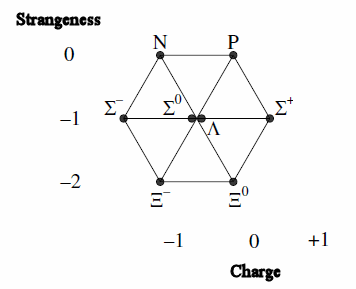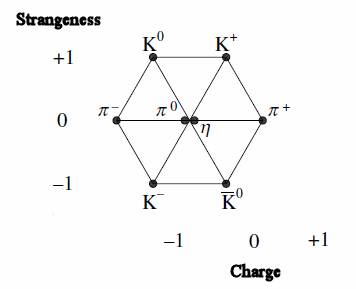Example patterns for some baryons and mesons (The Eightfold Way)

After finding the patterns an explanation was needed.  In 1964 Gell-Mann published a short article showing that the patterns could be produced if the known particles were viewed as combinations of 3 fundamental subunits with fractional charge, the up, down, and strange quarks and their antiquarks.  There were however problems with the Pauli Exclusion Principle.  The quarks are spin 1/2 fermions and the Δ++(uuu) and the Ω-(sss) seemed to contain at least two quarks with exactly the same quantum numbers.  The quark theory was not really accepted until deep inelastic scattering experiments revealed structure inside the protons in the later 1960s.

The charm quark was discovered in 1974, the bottom quark in 1977, and the top quark in 1995.  The tau particle was detected in a series of experiments between 1974 and 1977 and the discovery of the tau neutrino was announced in 2000.  It was the last of the particles in the Standard Model of elementary particles to be detected.

One of the current frontiers in the study of elementary particles concerns the interface between that discipline and cosmology.  The known quarks and leptons, for instance, are typically grouped in three families, where each family contains two quarks and two leptons.  Investigators have wondered whether additional families of elementary particles might be found.  Recent work in cosmology pertaining to the evolution of the universe has suggested that there could be no more families than four, and the cosmological theory has been substantiated by experimental work at the Stanford Linear Accelerator and at CERN, which indicates that there are no families of elementary particles other than the three that are known today.  For example, detailed studies of Z0 decays at CERN revealed that there can be no more than three different kinds of neutrino. If there was a fourth, or a fifth, further decay routes would be open to the Z0 which would affect its measured lifetime.

Conservation Laws

Leptons carry an additive quantum number called the lepton number L.  The leptons listed in the table above carry L = 1 and their antiparticles carry L = -1.   The electron number carried by electrons and electron neutrinos seems to an additive quantum number which is conserved in interactions.  Electrons and electron neutrinos have electron number 1 and positrons and electron anti-neutrinos carry electron number -1.  Muons, or μ-mesons, behave similarly as electrons.  They only have electromagnetic and weak interactions.  Only their mass, 106 MeV/c2, distinguishes them from electrons.  Along with νμ they carry an additive quantum number, the muon number, that which also seems to be conserved in interactions.  Muons decay as

μ+ --> e+ + νμ + νe

in about 10-6s.   The tau and its neutrino carry an additive quantum number as well, which seems to be conserved in interactions.  We say that the lepton family number LF also seems to be conserved. However if neutrinos have mass and can change flavor, for example, if muon neutrinos can change into electron neutrinos and vice versa, then only L is conserved.

Are there more such additive quantum numbers?  Yes, there is a group of particles called baryons, and a corresponding conserved quantum number called baryon number B.   Baryons have baryon number B = 1 and anti-baryons have baryon number B = -1.  The lightest baryon is the proton, and it is the only stable baryon. Since the neutron decays by n --> p + e - + νe and the electron and anti-neutrino are leptons, not baryons, B conservation requires that the neutron is also baryon.

It is fairly easy to spot a baryon in a table of elementary particles.  Suppose you are looking at a particle which might be a baryon.  If it is not the proton and it is a baryon, it must decay.  Baryon conservation then requires a baryon among the decay products, although you may not know which of the decay products is the baryon.  Let all of the decay products themselves decay.  The baryon's decay yields another baryon.  Keep going until all the particles are stable.  Among all the resulting particles there must be one net baryon.  Since the proton is the only stable baryon, that baryon must be a proton.  Hence, a particle is a baryon if and only if there is one net proton among its ultimate decay products.

Baryons are made up of 3 quarks.  All quarks have baryon number B = 1/3, and all anti-quarks have baryon number B = -1/3.

Everything else in the table besides the baryons and leptons is called a meson.  Mesons are made up of a quark and an anti-quark.  Mesons have L = 0 and B = 0, and they have no net leptons or baryons in their ultimate decay products.  The number of mesons is not conserved, so there is no "meson number."

After the discovery of pions other particles were discovered in rapid pace.  K-mesons (m = 494 MeV/c2) are similar to π -mesons, they do not carry a nucleon number.  The K-meson decays in the following way.

K+ --> π+ + π0

Hyperons are different.  The lightest hyperon is the Λ0 with m = 1116 MeV/c2.  It decays in the following way

Λ0 --> p + π-, or n + π0

A hyperons is a baryon.

As better accelerators became available the reaction below was observed

π+ + n --> Λ0 + K+

Obviously in the above process baryon number is conserved.  It was remarkable that reactions like

π+ + n --> Λ0 + π +

were never observed.  This prompted the introduction of a new quantum number, strangeness, by Gell-Mann and Pais.  The strangeness S(Λ0) = -1, S(K+) = 1.  Protons, neutron an pions have no strangeness.  In decay processes involving the strong interaction strangeness is conserved.  In decay processes involving the weak interaction, such as K-decay or hyperon decay, strangeness is not conserved.  This was the first case that some quantum numbers were conserved in strong interactions and electromagnetic interactions, but not in weak interactions.  Decay processes governed by the strong interaction can be distinguished easily from decay processes governed by the weak interaction.  Characteristic reaction times for the former are on the order of 10-23 s while for the latter they are on the order of 10-10 s.

Problems:

Determine whether a stationary proton can decay according to the scheme p --> π0 + π+.

• Solution:
We have to check whether the decay violates any of the conservation laws.
• Energy conservation:
Q = minitialc2 - mfinalc2 = mprotonc2 - (mπ0c2 + mπ+c2)
= (938.3 - (135.0 + 139.6))MeV = 663.7 MeV.
Energy conservation is not violated, the proton has enough mass to decay into the pair of pions.
• Momentum conservation:
Momentum can be conserved if the two pions move in opposite directions after the decay.
• Angular momentum conservation:
The proton is a spin 1/2 particle (fermion), the pions are spin 0 particles (bosons).
The orbital angular momentum quantum number can only be an integer, so there is no way that angular momentum can be conserved.  The proposed decay cannot occur.
• The decay also violates conservation of Baryon number.  The proton has B = 1 and the pions both have B = 0.

A Xi-minus particle decays in the following way:  Ξ-  --> Λ0 + π-.  The lambda-zero and the pi-minus particles are both unstable.  The following processes occur in a cascade, until only relatively stable products remain.
Λ0  --> p + π-,  π-  --> μ- + νμ,  μ-  --> e- + νμ + νe.
(a)  Is the Xi-minus particle a lepton or a hadron?  If the latter, is it a baryon or a meson?
(b)  Does the decay process conserve the three lepton numbers?

• Solution:
(a)  Only three families of leptons exist and none includes the the Xi-minus.  It must be a hadron.
The final decay products are p + 2(e-νμ + νμ + νe), since two pi-minus particles decay.  The baryon number of the proton is 1 and the baryon number of all the other particles is zero.  The net baryon number therefore is 1 and the Xi-minus is a baryon.
(b)  The proton has lepton number zero, the electron has electron lepton number 1, the anti-electron neutrino has electron lepton number -1, the muon neutrino has muon lepton number 1, and its antiparticle has muon lepton number -1.  All lepton numbers add up to zero after the decay, and they are zero before the decay, since the Xi-minus is a baryon.  The decay process conserves the three lepton numbers.

The Xi-minus particle has spin quantum number s = 1/2, charge q = -e, and strangeness quantum number S = -2.  It does not contain a bottom quark.  What combination of quarks is it made of?

• Solution:
The Xi-minus particle is a baryon, it is made up of three quarks.  It must contain two strange quarks to have S = -2.  This yields a charge of -(2/3)e.  We must add another quark with S = 0 and charge -(1/3)e.  Since the b-quark is excluded, we must add a d-quark.  The quark combination for the Xi-minus is dss.